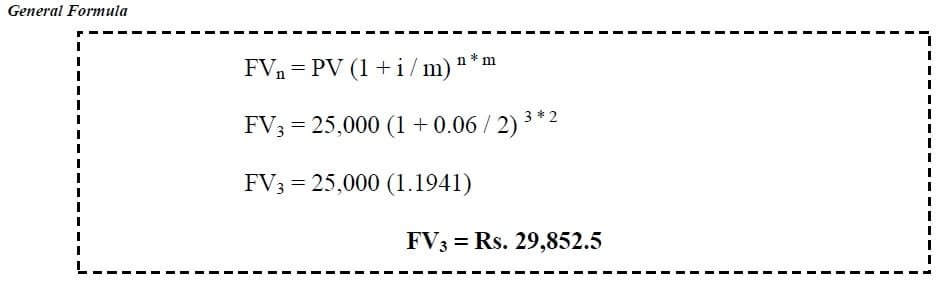Select Page

The future value of a single amount (one time cash flow) at some future time evaluated at a given interest rate assuming that compounding take place one time in a year (annually). Two methods for calculation: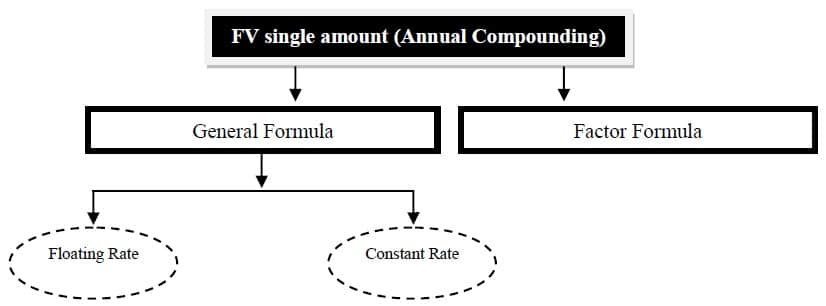## Formula Future Value of a Single Amount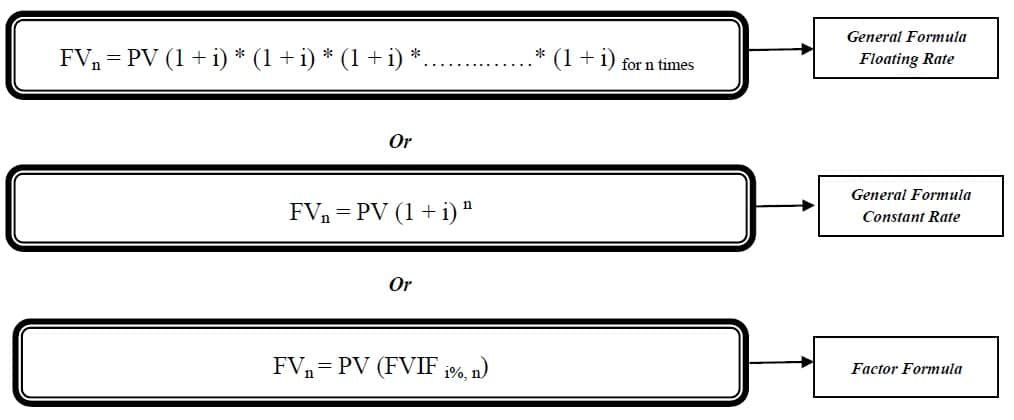FVn = Future value of n years

PV = Present value

i = Interest rate or Compound rate

n = Number of years

FVIF = Future Value Interest Factor

## Example # 1:

Ms Nosheen puts Rs. 10,000 in a savings account today, that pays 5% compounded annually. How much will she have in her account after ten years; by above mentioned three equations?

### Solution: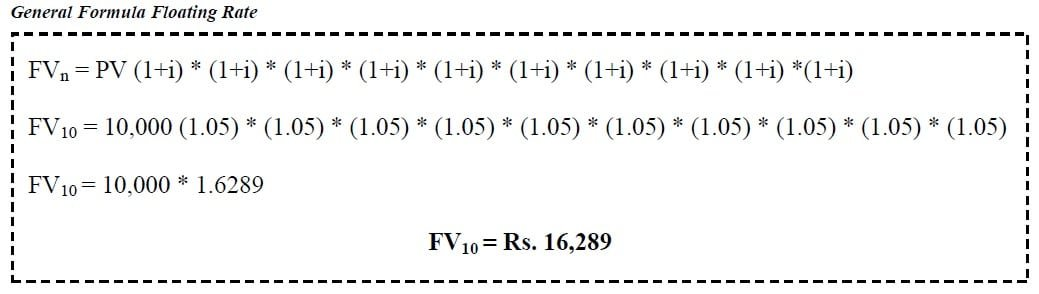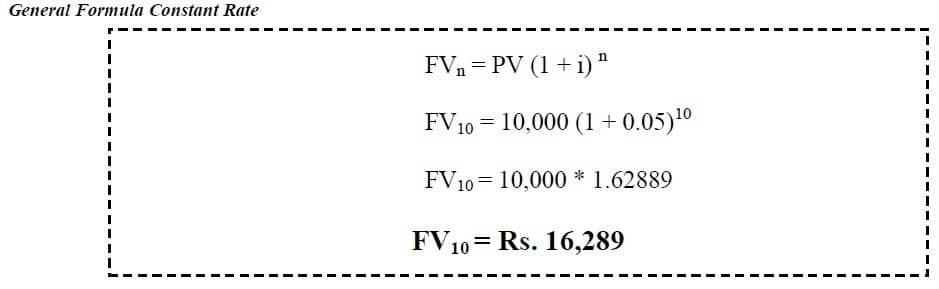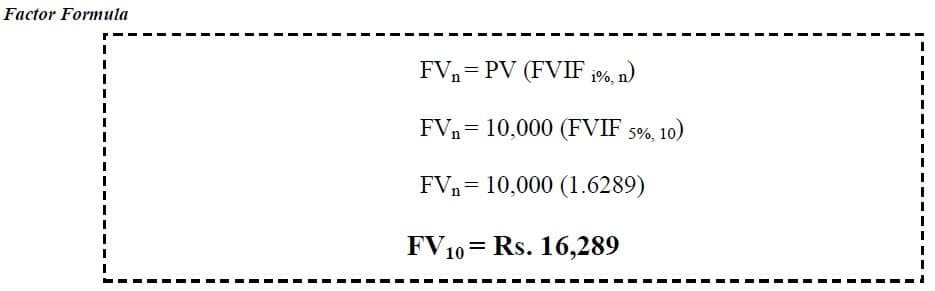## Example # 2:

Find the value of Rs. 10,000 today at the end of 10 periods at 5% per period by using scientific calculator and by Excel sheet?

### Solution: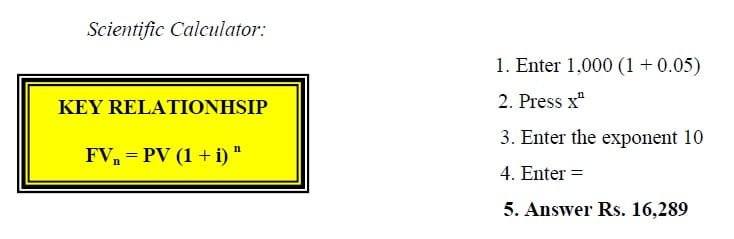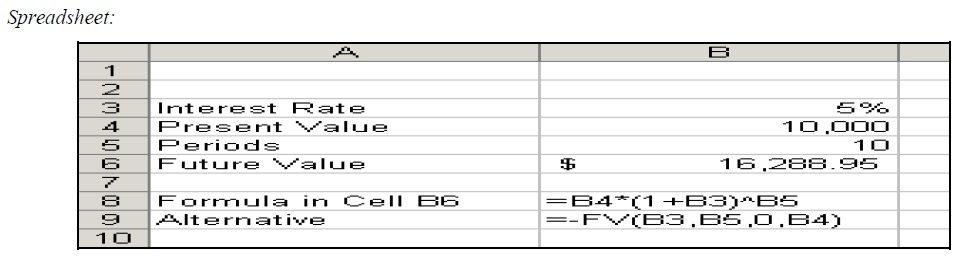>> Practice Future Value of a Single Amount MCQs

## Future value of a single amount (Intra-year Compounding)

The value of a single amount at some future time evaluated at a given interest rate assuming that compounding take place more than one time in a year (Intra-year). Interest rate reduced while periods of time increase by frequency of compounding (m) i.e. i/m and n*m. Two methods for calculation: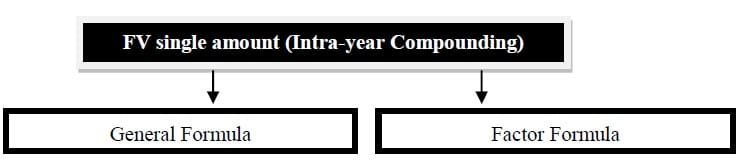## Formula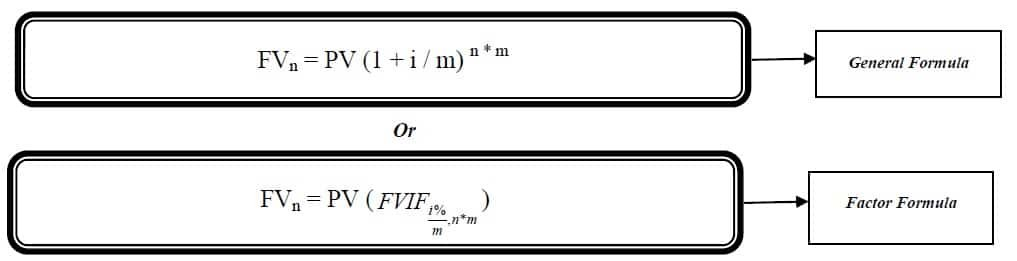FVn = Future value of n years

PV = Present value

i = Interest rate or Compound rate

m = Frequency of compounding

n = Number of years

FVIF = Future Value Interest Factor

>>> Practice Future Value of a Single Amount MCQs

Example # 3:

Mr. Naveed purchase a 3-year, 6-percent savings certificate for Rs. 25,000.  If interest is compounded semi-an­nually, what will be the value of the certificate when it matures by above mentioned two equations?

Solution: